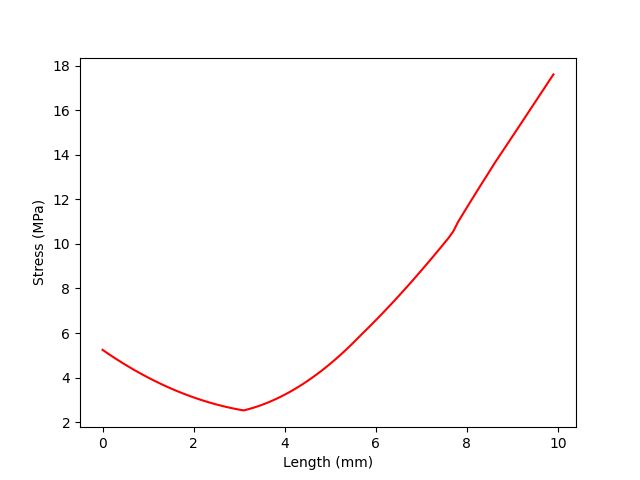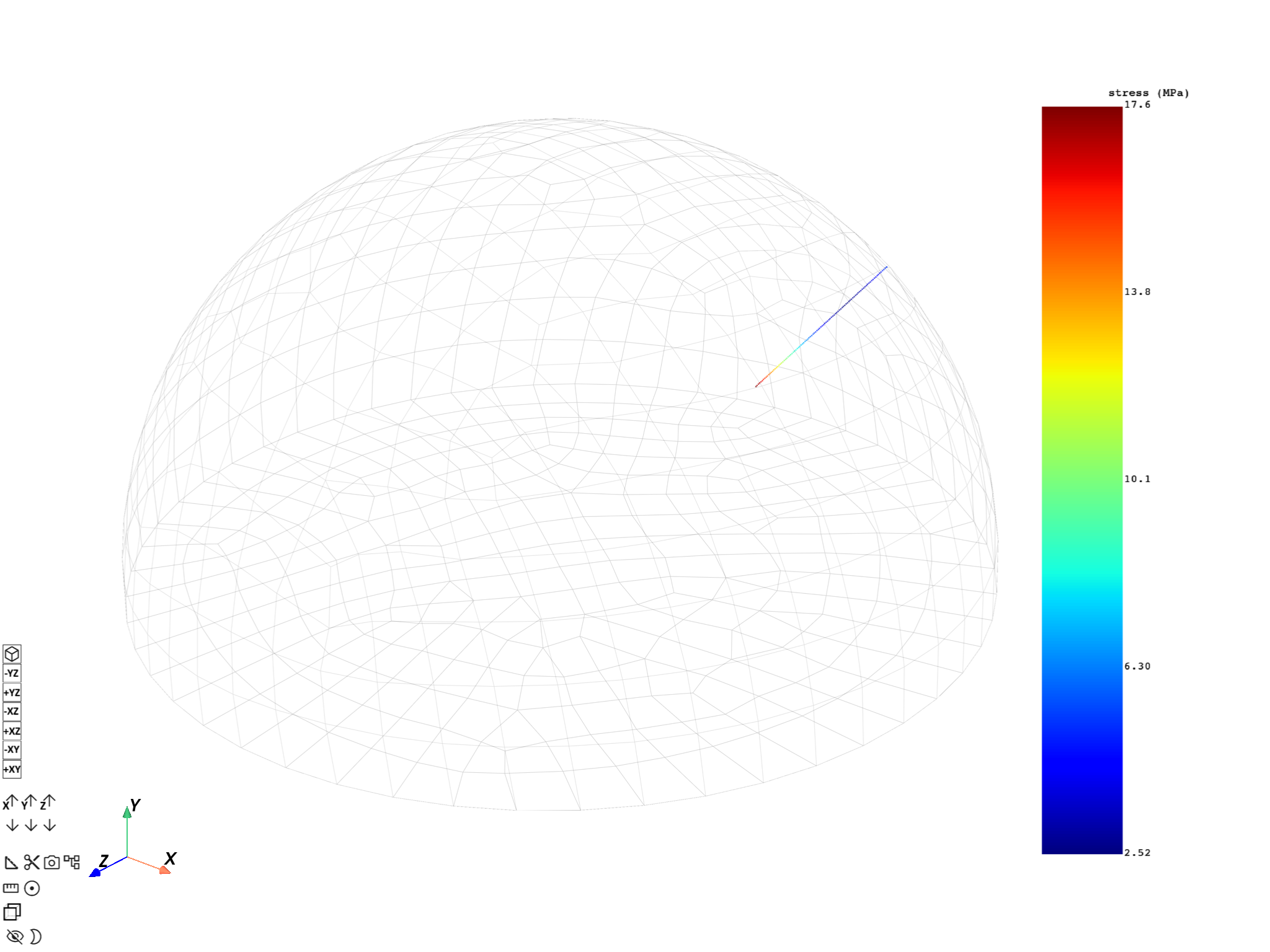# Stress gradient normal to a defined node#

This example shows how to plot a stress gradient normal to a selected node. Because the example is based on creating a path along the normal, the selected node must be on the surface of the geometry. A path is created of a defined length.

Import the DPF-Core module as `dpf` and import the included examples file and `DpfPlotter`.

```import matplotlib.pyplot as plt

from ansys.dpf import core as dpf
from ansys.dpf.core import examples
from ansys.dpf.core import operators as ops
from ansys.dpf.core.plotter import DpfPlotter
```

Open an example and print out the `Model` object. The `Model` class helps to organize access methods for the result by keeping track of the operators and data sources used by the result file.

Printing the model displays:

• Analysis type

• Available results

• Size of the mesh

• Number of results

• Unit

```path = examples.download_hemisphere()
model = dpf.Model(path)
print(model)
```
```DPF Model
------------------------------
Static analysis
Unit system: NMM: mm, ton, N, s, mA, degC
Physics Type: Mechanical
Available results:
-  displacement: Nodal Displacement
-  reaction_force: Nodal Force
-  stress: ElementalNodal Stress
-  elemental_volume: Elemental Volume
-  stiffness_matrix_energy: Elemental Energy-stiffness matrix
-  artificial_hourglass_energy: Elemental Hourglass Energy
-  thermal_dissipation_energy: Elemental thermal dissipation energy
-  kinetic_energy: Elemental Kinetic Energy
-  co_energy: Elemental co-energy
-  incremental_energy: Elemental incremental energy
-  elastic_strain: ElementalNodal Strain
-  structural_temperature: ElementalNodal Structural temperature
------------------------------
DPF  Meshed Region:
10741 nodes
3011 elements
Unit: mm
With solid (3D) elements
------------------------------
DPF  Time/Freq Support:
Number of sets: 1
1              1.000000       1              1
```

Define the node ID normal to plot the a stress gradient

```node_id = 1928
```

Print the mesh unit

```unit = model.metadata.meshed_region.unit
print("Unit: %s" % unit)
```
```Unit: mm
```

`depth` defines the length/depth that the path penetrates to. While defining `depth` make sure you use the correct mesh unit. `delta` defines distance between consecutive points on the path.

```depth = 10  # in mm
delta = 0.1  # in mm
```

Get the meshed region

```mesh = model.metadata.meshed_region
```

Get Equivalent stress fields container.

```stress_fc = model.results.stress().eqv().eval()
```

Define Nodal scoping. Make sure to define `"Nodal"` as the requested location, important for the `normals` operator.

```nodal_scoping = dpf.Scoping(location=dpf.locations.nodal)
nodal_scoping.ids = [node_id]
```

Get Skin Mesh because `normals` operator requires Shells as input.

```skin_mesh = ops.mesh.skin(mesh=mesh)
skin_meshed_region = skin_mesh.outputs.mesh.get_data()
```

Get normal at a node using `normals` operator.

```normal = ops.geo.normals()
normal.inputs.mesh.connect(skin_meshed_region)
normal.inputs.mesh_scoping.connect(nodal_scoping)
normal_vec_out_field = normal.outputs.field.get_data()
```

The normal vector is along the surface normal. You need to invert the vector using `scale` operator inwards in the geometry, to get the path direction.

```normal_vec_in_field = ops.math.scale(field=normal_vec_out_field, ponderation=-1.0)
normal_vec_in = normal_vec_in_field.outputs.field.get_data().data
```

Get nodal coordinates, they serve as the first point on the line.

```node = mesh.nodes.node_by_id(node_id)
line_fp = node.coordinates
```

Create 3D line equation.

```fx = lambda t: line_fp + normal_vec_in * t
fy = lambda t: line_fp + normal_vec_in * t
fz = lambda t: line_fp + normal_vec_in * t
```

Create coordinates using 3D line equation.

```coordinates = [[fx(t * delta), fy(t * delta), fz(t * delta)] for t in range(int(depth / delta))]
flat_coordinates = [entry for data in coordinates for entry in data]
```

Create field for coordinates of the path.

```field_coord = dpf.fields_factory.create_3d_vector_field(len(coordinates))
field_coord.data = flat_coordinates
field_coord.scoping.ids = list(range(1, len(coordinates) + 1))
```

Map results on the path.

```mapping_operator = ops.mapping.on_coordinates(
fields_container=stress_fc, coordinates=field_coord, create_support=True, mesh=mesh
)
fields_mapped = mapping_operator.outputs.fields_container()
```

Request the mapped field data and its mesh.

```field_m = fields_mapped
mesh_m = field_m.meshed_region
```

Create stress vs length chart.

```x_initial = 0.0
length = [x_initial + delta * index for index in range(len(field_m.data))]
plt.plot(length, field_m.data, "r")
plt.xlabel("Length (%s)" % mesh.unit)
plt.ylabel("Stress (%s)" % field_m.unit)
plt.show()
```Create a plot to add both meshes, `mesh_m` (the mapped mesh) and `mesh` (the original mesh)

```pl = DpfPlotter()